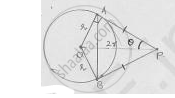# From a Point P, Two Tangents Pa and Pb Are Drawn to a Circle with Center O. If Op = Diameter of the Circle Shows that δApb is Equilateral. - Mathematics

#### Question

From a point P, two tangents PA and PB are drawn to a circle with center O. If OP =
diameter of the circle shows that ΔAPB is equilateral.

#### Solution

OP = 2r

Tangents drawn from external point to the circle are equal in length

PA = PBAt point of contact, tangent is perpendicular to radius.

In ΔAOP, sin 𝜃 ="opp.side"/"hypotenuse"=r/(2r)=1/2

𝜃 = 30°

∠APB = 20 = 60°, as PA = PB ∠BAP = ∠ABP = x.

In ΔPAB, by angle sum property

∠APB + ∠BAP + ∠ABP = 180°

2x = 120° ⇒ x = 60°

In this triangle all angles are equal to 60°

∴ ΔAPB is equilateral.

Is there an error in this question or solution?

#### APPEARS IN

RD Sharma Solution for Class 10 Maths (2018 (Latest))
Chapter 8: Circles
8.2 | Q: 17 | Page no. 35
RD Sharma Solution for Class 10 Maths (2018 (Latest))
Chapter 8: Circles
8.2 | Q: 17 | Page no. 35

#### Video TutorialsVIEW ALL 

From a Point P, Two Tangents Pa and Pb Are Drawn to a Circle with Center O. If Op = Diameter of the Circle Shows that δApb is Equilateral. Concept: Circles Examples and Solutions.